# Exercise 7.2 Cubes and Cube Roots- NCERT Solutions Class 8

## Chapter 7 Ex.7.2 Question 1

Find the cube root of each of the following numbers by prime factorization method.

(i) $$64$$

(ii) $$512$$

(iii) $$10648$$

(iv) $$27000$$

(v) $$15625$$

(vi) $$13824$$

(vii) $$110592$$

(viii) $$46656$$

(ix) $$175616$$

(x) $$91125$$

### Solution

Reasoning:

Factors in the prime factorization of cube should be grouped as triplets.

Steps:

(i)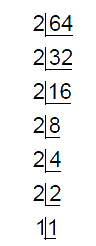\begin{align} 64&=\underline{2\times 2\times 2}\times \underline{2\times 2\times 2} \\ & ={{2}^{3}}\times {{2}^{3}} \\ \sqrt{64}&=2\times 2=4 \end{align}

(ii)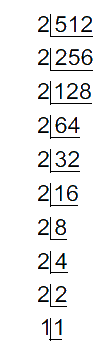\begin{align}512 =&\underline{2\times 2\times 2}\times \underline{2\times 2\times 2}\\&\times \underline{2\times 2\times 2} \\ & ={{2}^{3}}\times {{2}^{3}}\times {{2}^{3}} \\ \sqrt{512}&=2\times 2\times 2=8 \end{align}

(iii)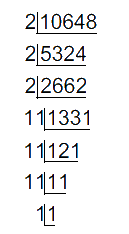\begin{align}10648&=\underline{2\times 2\times 2}\times \underline{11\times 11\times 11} \\ & ={{2}^{3}}\times {{11}^{3}} \\ \sqrt{10648}&=2\times 11=22 \end{align}

(iv)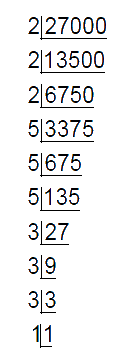\begin{align} 27000&=\begin{Bmatrix}\underline{2\times 2\times 2}\times \underline{3\times 3\times 3}\\\times \underline{5\times 5\times 5}\end{Bmatrix} \\ & ={{2}^{3}}\times {{3}^{3}}\times {{5}^{3}} \\ \sqrt{27000}&=2\times 3\times 5=30 \end{align}

(v)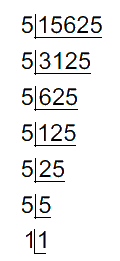\begin{align}15625&=\underline{5\times 5\times 5}\times \underline{5\times 5\times 5} \\ & ={{5}^{3}}\times {{5}^{3}} \\ \sqrt{15625}&=5\times 5=25 \end{align}

(vi)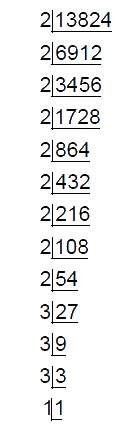\begin{align}13824&=\begin{Bmatrix}\underline{2\times 2\times 2}\times \underline{2\times 2\times 2}\\\times \underline{2\times 2\times 2}\times \underline{3\times 3\times 3}\end{Bmatrix} \\ & ={{2}^{3}}\times {{2}^{3}}\times {{2}^{3}}\times {{3}^{3}} \\ \sqrt{13824}&=2\times 2\times 2\times 3=24 \end{align}

(vii)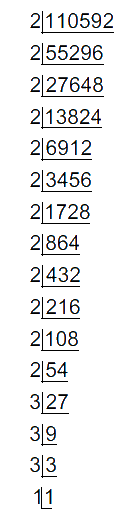\begin{align}110592&= \begin{Bmatrix} \underline{2\times 2\times 2}\times \underline{2\times 2\times 2}\\\times \underline{2\times 2\times 2}\times \underline{2\times 2\times 2}\\\times \underline{3\times 3\times 3}\end{Bmatrix} \\ & ={{2}^{3}}\times {{2}^{3}}\times {{2}^{3}}\times {{2}^{3}}\times {{3}^{3}} \\ \sqrt{110592}&=2\times 2\times 2\times 2\times 3=48 \end{align}

(viii)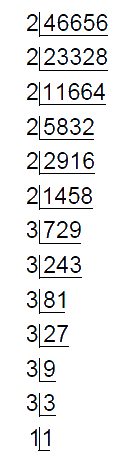\begin{align}46656& =\begin{Bmatrix}\underline{2\times 2\times 2}\times \underline{2\times 2\times 2}\\\times \underline{3\times 3\times 3}\times \underline{3\times 3\times 3} \end{Bmatrix}\\ & ={{2}^{3}}\times {{2}^{3}}\times {{2}^{3}}\times {{3}^{3}}\times {{3}^{3}} \\ \sqrt{46656} & =2\times 2\times 3\times 3=36 \end{align}

(ix)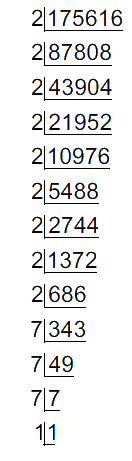\begin{align}175616&=\begin{Bmatrix}\underline{2\times 2\times 2}\times \underline{2\times 2\times 2}\\\times \underline{2\times 2\times 2}\times \underline{7\times 7\times 7} \end{Bmatrix}\\ & ={{2}^{3}}\times {{2}^{3}}\times {{2}^{3}}\times {{7}^{3}} \\ \sqrt{175616} &=2\times 2\times 2\times 7=56 \end{align}

(x)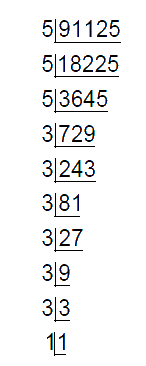\begin{align}91125&=\begin{Bmatrix}\underline{5\times 5\times 5}\times \underline{3\times 3\times 3}\\\times \underline{3\times 3\times 3}\end{Bmatrix} \\ & ={{5}^{3}}\times {{3}^{3}}\times {{3}^{3}} \\ \sqrt{91125}& =5\times 3\times 3=45 \end{align}

## Chapter 7 Ex.7.2 Question 2

State True or False:

(i). Cube of any odd number is even.

(ii). A perfect cube does not end with two zeros.

(iii). If square of a number ends with $$5,$$ then its cube ends with $$25$$.

(iv). There is no perfect cube which ends with $$8$$.

(v). The cube of a $$2$$-digit number may be a $$3$$-digit number.

(vi). The cube of a $$2$$-digit number may have seven or more digits.

(vii).The cube of a single digit number may be a single digit number.

### Solution

(i). Cube of any odd number is even:

Ans.  False

Reasoning:

Cubes of odd numbers are odd.

Cubes of even numbers are even.

(ii). A perfect cube does not end with two zeros:

Ans.  True

Reasoning:

Perfect cube may end with $$3$$ zeros (or) groups of $$3$$ zeros.

(iii). If square of a number ends with $$5,$$ then its cube ends with $$25$$

Ans.  False

Reasoning:

It is not always necessary that if the square of a number ends with $$5$$, then its cube will end with $$25$$.

For example, the square of $$5$$ is $$25$$ and $$25$$ has its unit digit as $$5$$. The cube of $$5$$ is $$125$$.

However, the square of $$15$$ is $$225$$ and also has its unit place digit as $$5$$ but the cube of $$15$$ is $$3375$$ which does not end with $$25$$.

(iv). There is no perfect cube which ends with $$8$$

Ans.  False

Reasoning:

The cubes of all the numbers having their unit place digit as $$2$$ will end with $$8$$.

The cube of $$12$$ is $$1728$$ and the cube of $$22$$ is $$10648$$.

(v). The cube of a $$2$$-digit number may be a $$3$$-digit number:

Ans.  False

Reasoning:

Cube of a $$1$$-digit number may have $$1$$-digit to $$3$$-digits.

Cube of a $$2$$-digit number may have $$4$$-digits to maximum $$6$$-digits

(vi). The cube of a $$2$$-digit number may have seven or more digits:

Ans.  False

Reasoning:

Cube of a $$1$$-digit number may have $$1$$-digit to $$3$$-digits.

Cube of a $$2$$-digit number may have $$4$$-digits to maximum $$6$$-digits.

(vii).The cube of a single digit number may be a single digit number:

Ans.  True

Reasoning:

Some examples

\begin{align}{1^3}&= 1\\{2^3}& = 8\end{align}

## Chapter 7 Ex.7.2 Question 3

You are told that $$1,331$$ is a perfect cube. Can you guess without factorization what its cube root is?

Similarly, guess the cube roots of $$4913,\; 12167, \;32768.$$

### Solution

Reasoning:

By grouping the digits of cube into $$3$$ and using Table 7.2

Steps:

(i) $$1331$$

Step 1:

$$1 =$$ Group $$2$$

$$331 =$$ Group $$1$$

Step 2: From group $$1$$, one’s digit of the cube root can be identified.

$$331=$$ One’s digit is $$1$$

Hence cube root’s one’s digit is $$1$$.

Step 3: From group $$2$$, which is $$1$$ only.

Hence cube root’s ten’s digit is $$1$$.

So we get  $$\sqrt[\mathrm{3}]{1331}=11$$

(ii) $$4913$$

Step 1:

$$4 =$$ Group $$2$$

$$913 =$$ Group $$1$$

Step 2: From group $$1$$, which is $$913$$.

$$913$$ $$=$$ One’s digit is $$3$$

We know that $$3$$ comes at the one’s place of a number only when it’s cube root ends in $$7$$. So, we get $$7$$ at the one’s place of the cube root. (Refer table 7.2 INFERENCE)

Step 3:From Group 2, which is 4.

\begin{align}{{1}^{3}}<4<{{2}^{3}}\end{align}

Taking lower limit. Therefore, ten’s digit of cube root is $$1$$.

So, we get .\begin{align}\sqrt[\mathrm{3}]{4913}=17\end{align}

(iii) $$12167$$

Step 1:

$$12 =$$ Group $$2$$

$$167 =$$ Group $$1$$

Step 2:  From group $$1$$, which is $$167$$.

$$167$$ $$=$$ One’s digit is $$7$$

We know that $$7$$ comes at the one’s place of a number only when it’s cube root ends in $$3$$. So, we get $$3$$ at the one’s place of the cube root. (Refer table 7.2 INFERENCE)

Step 3: From Group $$2$$, which is $$12$$

$${{2}^{3}}<12<{{3}^{3}}$$

Taking the lower limit. Therefore, ten’s digit of cube root is $$2$$.

So, we get .$$\sqrt[\mathrm{3}]{12167}=23$$

(iv) $$32768$$

Step 1:

$$32 =$$ Group $$2$$

$$768 =$$ Group $$1$$

Step 2: From group $$1$$, which is $$768$$.

$$768$$ $$=$$ One’s digit is $$8$$

We know that $$8$$ comes at the one’s place of a number only when it’s cube root ends in $$2$$. So, we get $$2$$ at the one’s place of the cube root. (Refer table 7.2 INFERENCE)

Step 3: From Group $$2$$, which is $$32$$.

$${{3}^{3}}<32<{{4}^{3}}$$

Taking lower limit. Therefore, ten’s digit of cube root is $$3$$.

So, we get .$$\sqrt[\mathrm{3}]{32768}=32$$

Instant doubt clearing with Cuemath Advanced Math Program